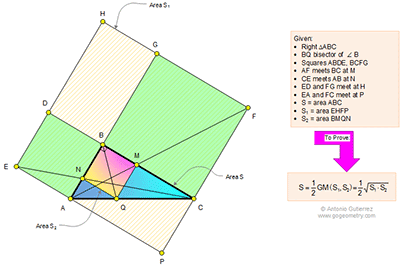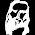## Monday, April 20, 2015

### Geometry Problem 1111: Right Triangle, External Squares, Cathetus, Angle Bisector, Area, Geometric Mean

Geometry Problem. Post your solution in the comment box below.
Level: Mathematics Education, High School, Honors Geometry, College.

Click the diagram below to enlarge it.#### 1 comment:

1.S = 1/2 ac
S₁ = (a+c)²
S₂ = (ac/(a+c))²

S₁S₂ = (ac)²
√(S₁S₂) = ac = 2S